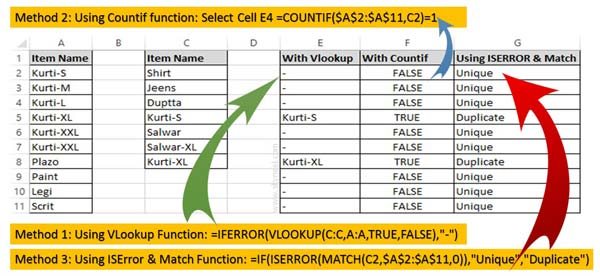# 3 Ways to find duplicate values on same worksheet – Excel

Excel allows you to search or find duplicate values on same worksheet. You can use given three methods to identify the duplicate values.

It is too much difficult to search duplicate values in active worksheet. In this guide we have to discuss 3 quick ways to find duplicate values on same worksheet. If you have more than one duplicate and unique records then you can easily find duplicate values on active worksheet.

Sometime you may need to identify duplicate value in active worksheet. If you try to find duplicate values manually then it will consume lot of time. But after reading this guide you can easily search duplicate values without any extra effort.## Excel – 3 Quick Ways to find duplicate values on same worksheet

Most of the users who are working on Excel are facing the problem how to search duplicate value or unique values in active worksheet. There is no need to worry you have to use given 3 quick ways to find duplicate values on same worksheet.

### Method 1: Find Duplicate Values with VLookup function

VLookup function searches a value in the extreme left column of the table_array and provides the value in the same row which is based on the index number.

= VLookup (lookup_value, table_array, col_index_num, [range])

Note: Before using the VLookup function, first you need to arrange first column of a table or array in the ascending order, otherwise VLookup function is not able to provide the accurate result.

Lookup_value: The value to be searched in the extreme first column of the table array.

Table_array: Two or more columns of data. Give cell reference of a range / range name.

Col_index_num: The column index_number in the table from which the searched value must be returned.

If col_index_num of returns 1 the value in the first column in table_array. If col_index_num is: Less than 1, this function returns an error value the #VALUE!. Sometime it is a greater than the number of columns in table_array, this function returns error value #REF!.

Range_lookup: It is an options part of this function. There are two options available in this part.

TRUE: Appropriate Match: This option work properly when first column of your table will be sorted in ascending order only otherwise it displays wrong
result.

False: Exact Match: VLookup by default check only on exact match method.

For E.g: Have a look and find any duplicate values on the same worksheet during applying VLookup formula in any sorted and unsorted table or database.

Step 1: Fill the required data in active worksheet.

Step 2: Type the following VLookup function in cell E2 =IFERROR(VLOOKUP(C:C,A:A,TRUE,FALSE),”-“) and press enter.

Step 3: Drag the VLookup function from range of cells E2:E11.

### Method 2: Search Duplicate Values with Countif function

Countif is an important function of Excel. You can easily count all those cells that meet criteria from given cells values in any worksheet.

Formula: COUNTIF (range, criteria)

range: It is the range of continuous cells which you want to evaluated for the specific condition.
criteria: It is the condition in the form of any number, expression or text value that define which cells to be counted.

Step 1: Type the following given countif function in cell F2 =COUNTIF(\$A\$2:\$A\$11,C2)=1 and press enter.

Step 2: Drag the VLookup function from range of cells F2:F11.

### Method 3: Identify Duplicate Values using ISERROR and Match function

ISError function is a built-in function in Excel. ISError function is used to checks whether a value is an error (#N/A, #VALUE!,#REF!, #DIV/0!, #NUM!, #NAME?, or #NULL!) and returns True or False.

ISERROR (Value)

Value: It is the value you want to test. Value can refer to a cell, formula or a name that refers to a cell, formula or value.

Match function is used to search specific value in the active worksheet. Now this function return the exact position of the value in the given range.

MATCH(lookup_value, lookup_array, [match_type])

lookup_value: Lookup values is the value which is search in the table array.

lookup_array: It is the range of cells in which you have to search lookup_value.

match_type: It’s an optional. The default value is 1. The number -1, 0, or 1.
1: Used to find the largest value that is less than or equal to lookup_value.
2: Used to find the first value that is exactly same to lookup_value.
-1: Used to find the smallest value that is greater than or equal to lookup_value.

Step 1: Type the following given function in cell G2 =IF(ISERROR(MATCH(C2,\$A\$2:\$A\$11,0)),”Unique”,”Duplicate”) and press enter.

Step 2: Drag the given function from range of cells G2:G11.

I hope after reading this guide you can use given quick Ways to find duplicate values on same worksheet in Excel. If you have any suggestion regarding his guide the please write us in the comment box. Thanks to all.